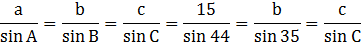### Sample ProblemUse the Law of Sines to solve this triangle. Round the lengths of the sides to three decimal places.

C= °

Side b= ft

Side c= ft

#### Solution

C=180°-35°-44°=101°Side b=15/sin 44 * (sin 35)=12.385

Side c=15/sin 44 * (sin 101)=21.197